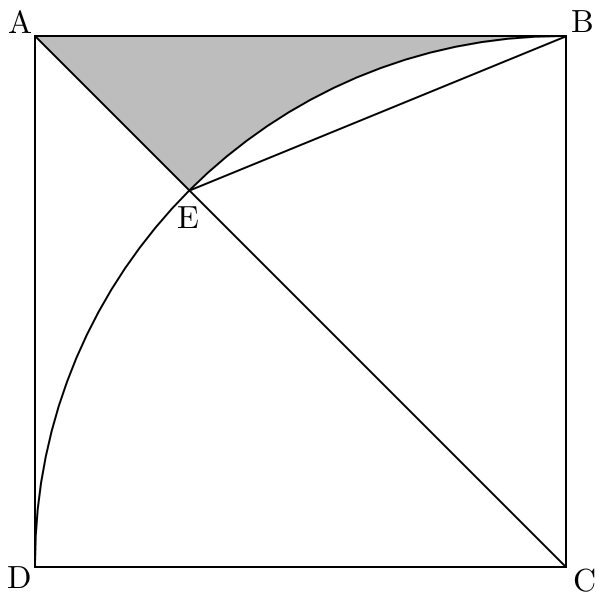# BE smart

Geometry Level 4A square $ABCD$ has an inscribed quadrant with centre $C$. Let $E$ be the point inside the square where the diagonal $AC$ and arc $BD$ intersect. The area of the shaded section is $121$.

Find the ceiling of the length of $BE$.

×